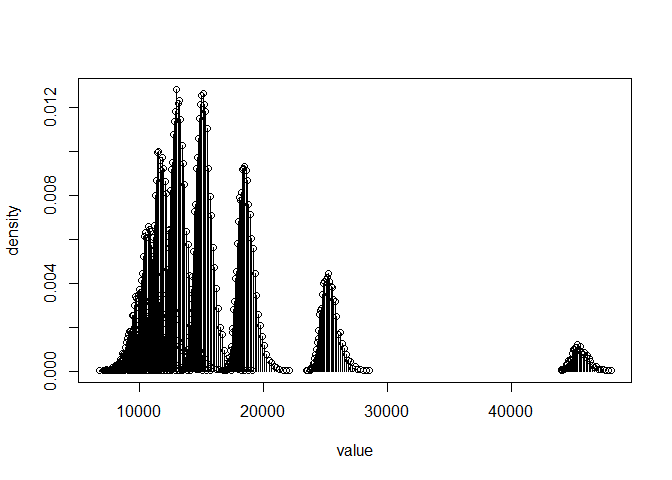# Estimating, Testing, and Simulating Abundance in a Mark-Recapture Experiment with recapr

#### 2021-09-07

Abundance Estimates and Standard Errors

Confidence Intervals

Simulation via Random Draws and Plotting Discrete Distributions

Hypothesis Testing and Power Calculation

Sample Size Recommendation

Tests of Consistency for the Estimator

Partially and Completely Stratified Estimators

## Abundance Estimates and Standard Errors

In a simple two-event mark-recapture experiment, point estimates of abundance can be calculated according to the Chapman, Petersen, or Bailey estimators using NChapman(), NPetersen(), or NBailey(), given the sample sizes in the first and second events, and the number of individuals recaptured in the second event. The Petersen estimator is the Maximum Likelihood Estimator (MLE), but the Chapman estimator generally performs better and is often recommended. Both assume sampling without replacement in the second sampling event. By contrast, the Bailey estimator assumes sampling with replacement in the second event.

The argument n1 denotes the sample size in the first event, with n2 denoting the sample size in the second event and m2 denoting the number of marked (recaptured) individuals in the second event.

library(recapr)
NChapman(n1=100, n2=150, m2=20)    # Abundance estimate
##  725.2381
vChapman(n1=100, n2=150, m2=20)    # estimated variance
##  16348.22
seChapman(n1=100, n2=150, m2=20)   # standard error
##  127.8601

## Confidence Intervals

Confidence intervals can be generated for the Chapman, Petersen, or Bailey estimators using ciChapman(), ciPetersen(), or ciBailey(). A point estimate is returned as well as two confidence intervals, one using a Wald-type Normal-distribution assumption, and the other calculated by means of bootstrapping capture histories. The bootstrapping interval is likely to have more appropriate bounds than the Normal interval, and has demonstrated better coverage in testing via simulation.

ciChapman(n1=100, n2=150, m2=20)
## $Nhat ##  725.2381 ## ##$ciNorm
##  474.6368 975.8394
##
## $ciBoot ##  524.8966 1172.1538 ## Simulation via Random Draws and Plotting Discrete Distributions Vectors of random draws can be generated for the Chapman, Petersen, or Bailey estimators using rChapman(), rPetersen(), or rBailey() from an assumed value of total abundance (N). This may be useful for simulation. Sample sizes n1 and n2 may be specified, but capture probabilities p1 and/or p2 may be used instead. If so, sample size(s) will first be drawn from a binomial distribution for each random draw before drawing from the abundance estimator. This will result in a greater degree of dispersion, but may be appropriate in some cases. A plotting function is also provided for vectors of discrete (non-continuous) data. Abundance estimates are calculated from count data, with the result having a non-integer but also non-continuous support. It is possible that plotdiscdensity() may be more appropriate for plotting a discrete (non-continuous) density than a kernel density plot or histogram, as the discontinuity is made explicit. draws <- rChapman(length=10000, N=1500, n1=100, n2=120) plotdiscdensity(draws)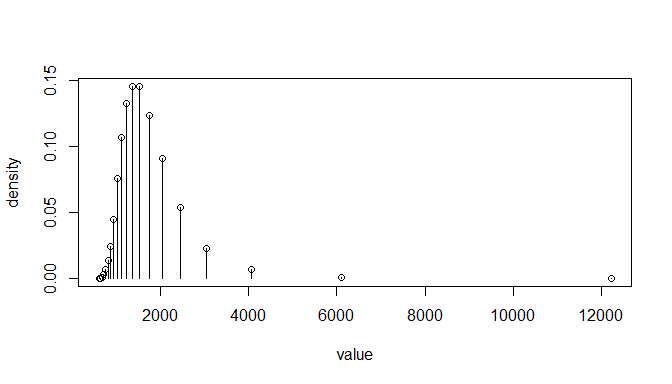## Hypothesis Testing and Power Calculation Approximate p-values can be returned using pChapman(), pPetersen(), or pBailey(), which use many random draws to simulate a null distribution. The null hypothesis abundance is specified in the nullN argument, along with sample sizes n1 and n2. The observed abundance estimate can be specified using estN, or else the number of recaptures can be used directly, as m2. The alternative hypothesis can be specified using the alternative argument, as alternative="less", "greater", or "2-sided". In the example given below, the null-hypothesis abundance is 500, with 100 individuals observed in the first and second events, with 28 recaptures in the second event. output <- pChapman(nullN=500, n1=100, n2=100, m2=28, alternative="less") output ##$estN
##  350.7586
##
## $pval ##  0.02068 plotdiscdensity(rChapman(length=100000, N=500, n1=100, n2=100)) # null distribution abline(v=500, lwd=2, lty=2) # Null hypothesis abundance plotted as a dashed line abline(v=output$estN, lwd=2, col=2)      # Observed (estimated) abundance plotted as a red line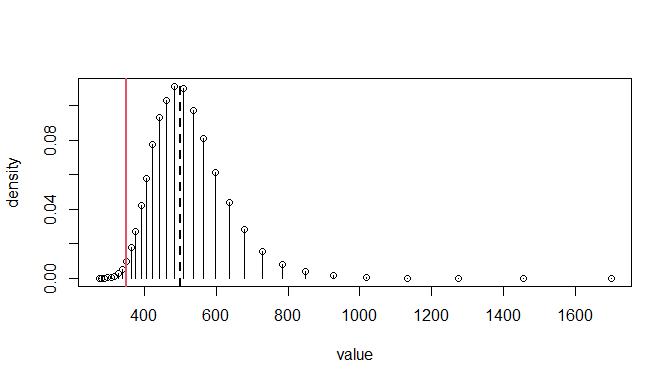Power calculation can be done with powChapman(), powPetersen(), or powBailey(), which use random draws from an assumed true (alternative) distribution, given the sample sizes of both events. The nullN argument specifies the abundance used by the null hypothesis, and the trueN argument specifies the assumed true abundance used for the power calculation. The n1 and n2 arguments give the sample sizes, and alternative gives the direction of the alternative hypothesis (defaults to "less"), with alpha specifying the level of the test to use.

In the example given below, the power is calculated for a one-tailed test of a null abundance of 500, assuming a true abundance of 400. The test powers are then calculated and plotted for assumed abundances from 250 to 450. If the true abundance is 325, a one-tailed test of $$H_0: N \geq 500$$ will have a power of roughly 90%.

powChapman(nullN=500, trueN=400, n1=100, n2=100, nsim=1000)
##  0.333
Ntotry <- seq(from=250, to=450, by=25)
power <- sapply(Ntotry, function(x)
powChapman(nullN=500, trueN=x, n1=100, n2=100, nsim=1000))
plot(Ntotry, power)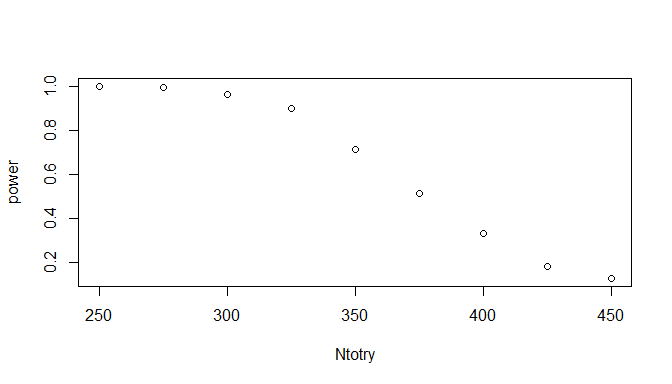## Sample Size Recommendation

Given a best-guess at the true abundance and possibly the sample size in one sampling event, a recommendation for the sample size(s) can be calculated from the desired confidence and relative accuracy with the methods of Robson & Regier (1964) using n2RR(). Desired estimate confidence and accuracy (elsewhere termed “precision”) of 95% and 10%, respectively, is analogous to estimation “such that the estimated abundance will be within 10% of the true value 95% of the time”.

Recommendations for sample size are provided for two scenarios: if the size of the other sample is as specified, and if the two sample sizes are equal, which is the most efficient for sampling in terms of total sample size ($$n_1+n_2$$). The example below gives the full output for all allowed values of confidence and relative accuracy.

n2RR(N=1000, n1=100)
## $conf_0.99 ## Rel acc n2 from specified n1 n if n1=n2 ## 1 0.50 305 181 ## 2 0.25 550 270 ## 3 0.20 639 308 ## 4 0.15 746 364 ## 5 0.10 863 455 ## 6 0.05 961 622 ## 7 0.01 999 891 ## ##$conf_0.95
##   Rel acc n2 from specified n1 n if n1=n2
## 1    0.50                  180        136
## 2    0.25                  387        210
## 3    0.20                  484        244
## 4    0.15                  617        298
## 5    0.10                  780        385
## 6    0.05                  933        555
## 7    0.01                  998        862
##
## $conf_0.9 ## Rel acc n2 from specified n1 n if n1=n2 ## 1 0.50 118 109 ## 2 0.25 293 177 ## 3 0.20 387 210 ## 4 0.15 525 260 ## 5 0.10 711 344 ## 6 0.05 908 511 ## 7 0.01 996 839 ## ##$conf_0.85
##   Rel acc n2 from specified n1 n if n1=n2
## 1    0.50                   81         90
## 2    0.25                  233        155
## 3    0.20                  320        186
## 4    0.15                  455        234
## 5    0.10                  652        314
## 6    0.05                  882        477
## 7    0.01                  995        820
##
## $conf_0.8 ## Rel acc n2 from specified n1 n if n1=n2 ## 1 0.50 57 76 ## 2 0.25 189 139 ## 3 0.20 268 168 ## 4 0.15 395 213 ## 5 0.10 596 289 ## 6 0.05 856 448 ## 7 0.01 994 803 ## ##$conf_0.75
##   Rel acc n2 from specified n1 n if n1=n2
## 1    0.50                   41         65
## 2    0.25                  155        125
## 3    0.20                  225        153
## 4    0.15                  343        195
## 5    0.10                  543        267
## 6    0.05                  827        422
## 7    0.01                  992        785

Output can be simplified by providing values of confidence and relative accuracy in the conf and acc arguments.

n2RR(N=1000, n1=100, conf=c(0.9,0.95), acc=c(0.15,0.1,0.05))
## $conf_0.9 ## Rel acc n2 from specified n1 n if n1=n2 ## 1 0.15 525 260 ## 2 0.10 711 344 ## 3 0.05 908 511 ## ##$conf_0.95
##   Rel acc n2 from specified n1 n if n1=n2
## 1    0.15                  617        298
## 2    0.10                  780        385
## 3    0.05                  933        555

An alternative approach using simulation is provided with plotn2sim(), in which the relative accuracy is simulated for a range of sample size values for the second event, at different levels of confidence. The values to plot for n2 can be set by n2range and n2step, giving the range endpoints and step size, respectively.

plotn2sim(N=1000, n1=100)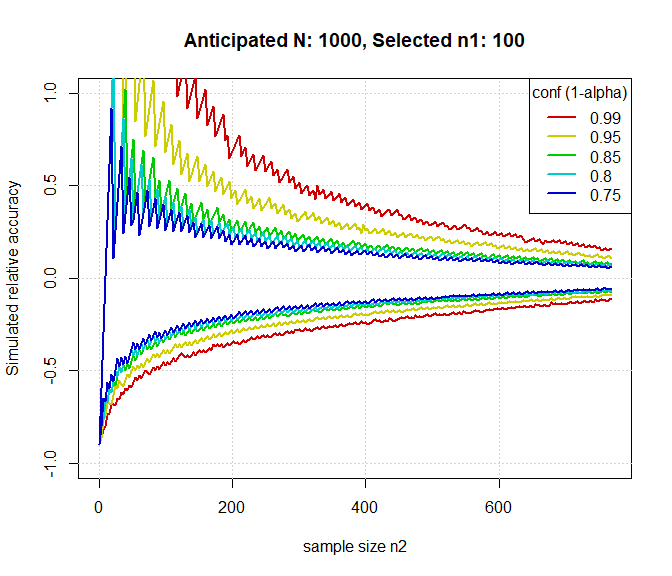Simulation is also used in plotn1n2simmatrix(), in which the relative accuracy is calculated for a range of sample size values for both sampling events for a given level of confidence, in this case 95%.

plotn1n2simmatrix(N=1000)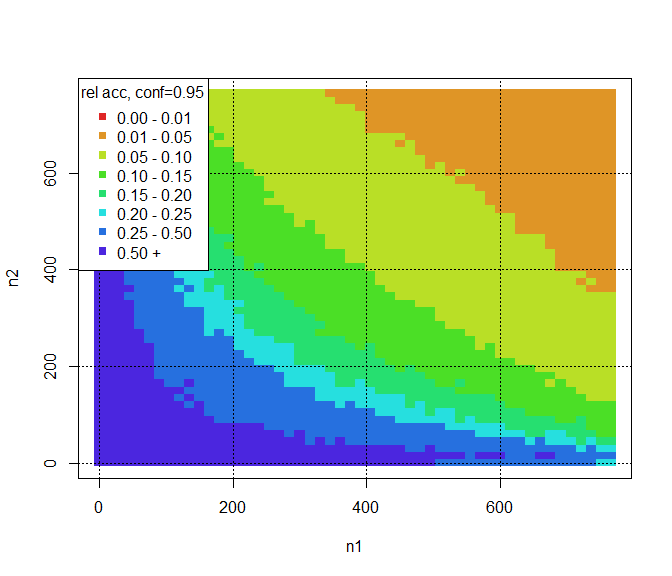## Tests of Consistency for the Estimator

### Testing the Need for Partial Stratification

For a simple Petersen-type estimator of abundance to be consistent, one of three conditions must be true: either there must be complete mixing in the time between events, or the probability of capture must be equal for all individuals in the first event, or the probability of capture must be equal for all individuals in the second event. This is typically investigated by means of a set of $$\chi^2$$ tests for each condition, with failure to reject the null hypothesis of any of the tests indicating that a simple Petersen-type estimator is reasonable to use. If the null hypotheses are rejected in all tests, a completely stratified or partially stratified (Darroch-type) estimator should be used.

The consistencytest() function is provided for the instance that sampling in both events is stratified in such a way that movement between strata may occur, such as spatial or temporal stratification. The stratification used in events 1 and 2 do not need to be the same. Arguments n1 and n2 accept vectors of sample sizes in each event by strata. Recaptures of marked individuals can be specified in one of two ways: either as vectors of strata membership in m2strata1 and m2strata2, or as a matrix in stratamat. Arguments m2strata1 and m2strata2 accept vectors of the first- and second-event stratum membership for each recaptured individual, with only entries of 1, 2, 3, ... accepted. Alternatively, stratamat accepts a matrix in which each cell represents the number of recaptures for each combination of event 1 and event 2 strata, with rows corresponding to event 1 strata and columns corresponding to event 2 strata.

In the example below, there were two strata in event 1 (with sample sizes of 284 and 199) and three strata in event 2, and 30 individuals were marked in stratum 1 of event 1 and were recaptured in stratum 1 of event 2. These tests yield strong evidence of incomplete mixing in the time between events and of unequal marked to unmarked ratios among recapture strata, but also no evidence against the probability of re-sighting a released animal being independent of its stratum of origin. Therefore, use of a simple Petersen-type estimator may be considered justified if closure is assumed.

mat <- matrix(c(30,15,1,0,22,15), nrow=2, ncol=3, byrow=TRUE)
consistencytest(n1=c(284,199), n2=c(347,3616,1489), stratamat=mat)
## MIXING TEST
## H0: Movement probabilities from stratum i to stratum j are the same among sections (all theta_ij = theta_j)
##
##    1  2  3 not recaptured
## 1 30 15  1            238
## 2  0 22 15            162
##
##  X-squared:  44.43179   df:  3   p-val:  1.221827e-09
##
## EQUAL PROPORTIONS TEST (SPAS terminology)
## H0: Marked to unmarked ratio among recapture strata is constant (Sum_i a_i*theta_ij/U_j = k)
##
##              1    2    3
## recaptured  30   37   16
## unmarked   317 3579 1473
##
##  X-squared:  125.4408   df:  2   p-val:  5.766008e-28
##
## COMPLETE MIXING TEST (SPAS terminology)
## H0: Probability of re-sighting a released animal is independent of its stratum of origin (Sum_i theta_ij*p_j = d)
##
##                  1   2
## recaptured      46  37
## not recaptured 238 162
##
##  X-squared:  0.3185941   df:  1   p-val:  0.5724538
## 

Since the decision to use a simple Petersen-type estimator is based on a failure to reject, the power of the tests used must be considered. The powconsistencytest() function uses simulation as well as Cohen’s non-central $$\chi^2$$ method to calculate the power of the consistency tests, given anticipated values of sample sizes by strata and assumed movement probabilities.

The pmat argument is a matrix of assumed movement probabilities between strata, with rows corresponding to first-event strata and columns corresponding to second-event strata, with an additional column corresponding to the probability of NOT being recaptured in the second event. Values are conditioned on row, that is, by first-event strata. For example, a pmat with a first row equal to (0.05, 0.1, 0.15, 0.7) would imply a 5% chance that individuals captured in the first-event strata 1 will be recaptured in second-event strata 1, and a 70% chance that individuals captured in the first-event strata 1 will not be recaptured in event 2.

Because of the row-wise scaling, specifying a row equal to (0.05,0.1, 0.15, 0.7) would be equivalent to that row having values (1, 2, 3, 14).

mat <- matrix(c(1,2,3,10,3,2,1,10), nrow=2, ncol=4, byrow=TRUE)
powconsistencytest(n1=c(100,200), n2=c(100,100,100), pmat=mat)
## MIXING TEST
## H0: Movement probabilities from stratum i to stratum j are the same among sections (all theta_ij = theta_j)
## Sample size (first event):  300    Significance level:  0.05
##
##  Null hypothesis cross-classification probabilities:
##        1      2      3 Not recap
## 1 0.0486 0.0417 0.0347    0.2083
## 2 0.0972 0.0833 0.0694    0.4167
##
##  Alternative hypothesis cross-classification probabilities:
##        1      2      3 Not recap
## 1 0.0208 0.0417 0.0625    0.2083
## 2 0.1250 0.0833 0.0417    0.4167
##
##  Null hypothesis expected counts:
##       1    2     3 Not recap
## 1 14.58 12.5 10.42      62.5
## 2 29.17 25.0 20.83     125.0
##
##  Alternative hypothesis expected counts:
##       1    2     3 Not recap
## 1  6.25 12.5 18.75      62.5
## 2 37.50 25.0 12.50     125.0
##
## Power:  0.9496757    Power (from simulation):  0.9541
##
##
## EQUAL PROPORTIONS TEST
## H0: Equal probability of capture among n1 strata (all p_i equal)
## Sample size (second event):  300    Significance level:  0.05
##
##  Null hypothesis capture probabilities:
##                 1      2      3
## Recaptured 0.1250 0.1250 0.1250
## Unmarked   0.2083 0.2083 0.2083
##
##  Alternative hypothesis capture probabilities:
##                 1      2      3
## Recaptured 0.1458 0.1250 0.1042
## Unmarked   0.1875 0.2083 0.2292
##
##  Null hypothesis expected counts:
##               1    2    3
## Recaptured 37.5 37.5 37.5
## Unmarked   62.5 62.5 62.5
##
##  Alternative hypothesis expected counts:
##                1    2     3
## Recaptured 43.75 37.5 31.25
## Unmarked   56.25 62.5 68.75
##
## Power:  0.3532643    Power (from simulation):  0.3515
##
##
## COMPLETE MIXING TEST
## H0: Equal probability of recapture among n2 strata (all p_j equal)
## Sample size (first event):  300    Significance level:  0.05
##
##  Null hypothesis capture probabilities:
##               1      2
## Marked   0.1250 0.2500
## Unmarked 0.2083 0.4167
##
##  Alternative hypothesis recapture probabilities:
##               1      2
## Marked   0.1250 0.2500
## Unmarked 0.2083 0.4167
##
##  Null hypothesis expected counts:
##             1   2
## Marked   37.5  75
## Unmarked 62.5 125
##
##  Alternative hypothesis expected counts:
##             1   2
## Marked   37.5  75
## Unmarked 62.5 125
##
## Power:  0.05    Power (from simulation):  0.0526
##
## 

### Testing the Need for Complete Stratification

In the event that there is no movement observed between strata, or the population is stratified in such a way that there can be no movement between strata (such as by age or sex), a stratified estimator may be needed. The strattest() function provides a means of conducting the typical $$\chi^2$$ tests to investigate the necessity of a stratified estimator.

Since strata membership remains the same in both events, usage is simpler, with n1, n2, and m2 accepting vectors of first- and second-event sample sizes and recapture numbers by strata. In the example below, there is strong evidence of unequal capture probabilities in the first event but not in the second, and very strong evidence of differences in respective stratum capture probabilities between the first and second events.

strattest(n1=c(100,100), n2=c(50,200), m2=c(20,15))
##
##  Equal proportions test
## H0: Equal probability of capture among n1 strata (all p_i equal)
##
##             1   2
## recaptured 20  15
## unmarked   30 185
##
##  X-squared:  32.44394   df:  1   p-val:  1.226811e-08
##
##
##  Complete mixing test
## H0: Equal probability of recapture among n2 strata (all p_j equal)
##
##                 1  2
## recaptured     20 15
## not recaptured 80 85
##
##  X-squared:  0.5541126   df:  1   p-val:  0.4566422
##
##
##  First vs. second event test
## H0: Probabilities of capture are the same between first and second events (all p_i equal to respective p_j)
##
##                1   2
## first event  100 100
## second event  50 200
##
##  X-squared:  43.66013   df:  1   p-val:  3.906508e-11
## 

Similarly, the powstrattest() function provides power estimates for the tests used in strattest(), given assumed values of total abundance by strata and anticipated values of sample sizes by strata, using simulation as well as Cohen’s non-central $$\chi^2$$ method.

powstrattest(N=c(1000,2000), n1=c(100,200), n2=c(200,200))
## $Test1 ## First-event capture probabilities ## ## Detecting capture probabilities for each strata: 0.1 0.1 ## As compared to null values: 0.1 0.1 ## sample size: 400 and significance level: 0.05 ## ## power: 0.05 ## power (from simulation): 0.04948 ## ##$Test2
## Second-event capture probabilities
##
## Detecting capture probabilities for each strata:  0.2 0.1
## As compared to null values:  0.1333333 0.1333333
## sample size:  300  and significance level:  0.05
##
## power:  0.6707468
## power (from simulation):  0.65945
##
## $Test3 ## First-event vs. second-event capture probabilities ## ## Detecting capture probabilities for each strata: 0.6666667 0.5 ## As compared to null values: 0.5714286 0.5714286 ## sample size: 700 and significance level: 0.05 ## ## power: 0.9928497 ## power (from simulation): 0.99563 ## Partially and Completely Stratified Estimators If sampling is stratified in both sampling events and some movement exists between strata but mixing is incomplete in the time between events and tests indicate unequal capture probabilities in both events, a partially stratified (Darroch-type) estimator should be used. The NDarroch() function provides a means for doing this, and argument usage is the same as that in consistencytest(). The function returns abundance estimates and standard errors for each stratum in both sampling events, as well as an overall abundance estimate and standard error. Implementation is currently using Darroch’s method for calculations, and will only accept non-singular input matrices. mat <- matrix(c(59,30,1,45,280,38,0,42,25), nrow=3, ncol=3, byrow=TRUE) NDarroch(n1=c(484,1468,399), n2=c(847,6616,2489), stratamat=mat) ##$Nhat_strata1
##   3584.554 16769.123 33699.792
##
## $se_Nhat_strata1 ##  1914.125 6902.991 8979.480 ## ##$Nhat_strata2
##   6360.409 18069.681 29623.380
##
## $se_Nhat_strata2 ##  848.4842 4331.6190 7791.7721 ## ##$Nhat
##  54053.47
##
## $se_Nhat ##  4890.999 If no movement can occur between strata, a completely stratified estimator can be used. Functions Nstrat(), vstrat(), sestrat(), and cistrat(), are provided to compute abundance estimates, estimated variance, standard error, and confidence intervals. In all cases, n1, n2, and m2 accept vectors of first- and second-event sample sizes and recapture numbers by strata, and the additional estimator accepts the type of Petersen-type estimator to use: either "Chapman", "Petersen", or "Bailey". Additionally, rstrat() generates random draws from given vectors of N, n1, and n2, or with vectors of capture probabilities p1 and/or p2. Nstrat(n1=c(100,200), n2=c(100,500), m2=c(10,10)) ##  10080 vstrat(n1=c(100,200), n2=c(100,500), m2=c(10,10)) ##  6513699 sestrat(n1=c(100,200), n2=c(100,500), m2=c(10,10)) ##  2552.195 cistrat(n1=c(100,200), n2=c(100,500), m2=c(10,10)) ##$Nstrat
##  10080
##
## $Nhat_by_strat ##  926.3636 9153.6364 ## ##$ciNorm_strat
##   5077.79 15082.21
##
## \$ciBoot_strat
##   6649.363 20866.843
draws <- rstrat(length=10000, N=c(500,1000), n1=c(100,200), n2=c(100,500))
plotdiscdensity(draws)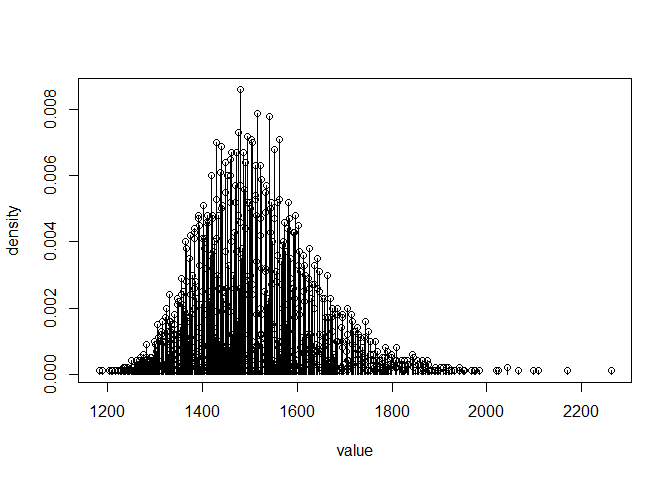draws <- rstrat(length=100000, N=c(5000,10000), n1=c(500,200), n2=c(500,200))
plotdiscdensity(draws)